# Basic Arithmetic : Ratio Tables

## Example Questions

### Example Question #1 : Ratio Tables

Find the value ofin the following ratio table: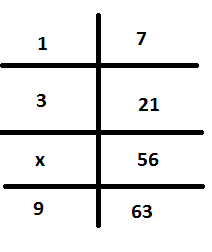Explanation:

The numbers on the left side to the numbers on the right side are in a ratio of 1 to 7. In other words, multiply the numbers on the left by 7 to get the numbers on the right.

Using that ratio, we arrive at the following equation for x: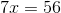Now, divide both sides by 7.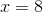### Example Question #1 : Ratio TablesFind the value ofin the ratio table above.Explanation:

To find, we can set up the following ratios: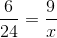Now, cross-multiply and solve.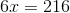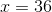### Example Question #2 : Ratio TablesFind the value ofin the ratio table above.Explanation:

To find the multiplier, divide two numbers from the same row: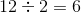Now, multiply this byand set that equal to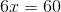Divide both sides by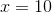### Example Question #3 : Ratio Tables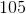In the ratio table above, find.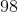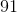Explanation:

To figure out the ratio, divide the second column by the first.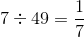Since we are multiplying the first column by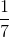to get the second column, we can set up the following equation for.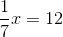Multiply both sides by 7.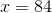### Example Question #4 : Ratio TablesFind the value ofin the ratio table above.Explanation:

To find, we can set up the following ratio: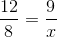Now, cross multiply and solve.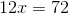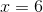### All Basic Arithmetic Resources